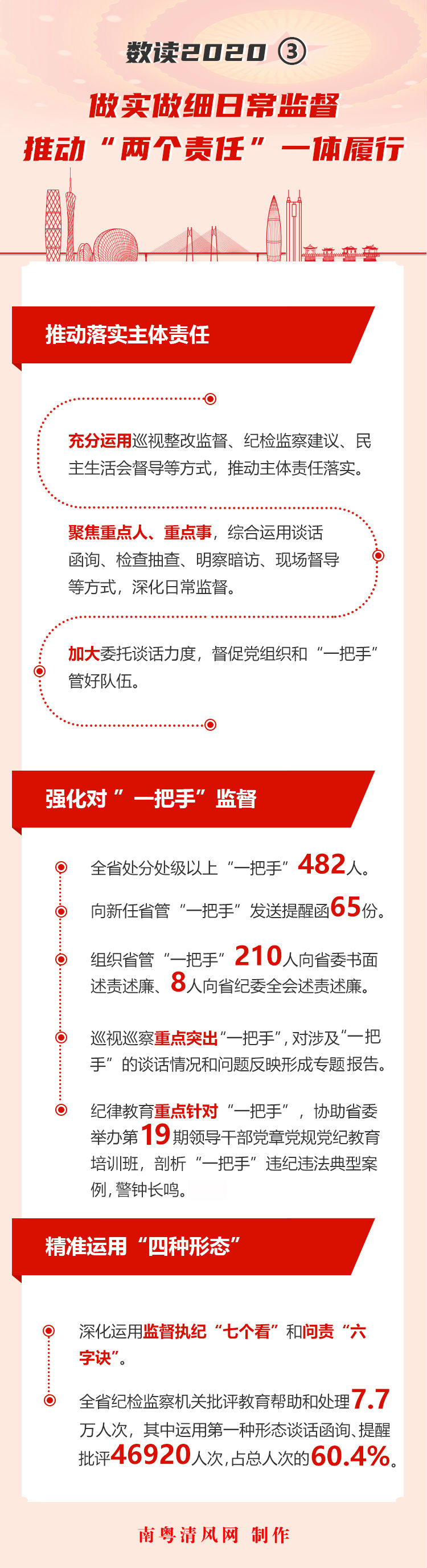## 数读2020③｜做实做细日常监督 推动“两个责任”一体履行

### 来源：南粤清风网 发布时间：2021-03-20 12:55 分享 QQ空间 新浪微博 QQ 微信 window._bd_share_config={"common":{"bdSnsKey":{"tsina":"2078561600 ","tqq":"801099517"},"bdText":"","bdMini":"1","bdMiniList":["qzone","tsina","tqq","sqq","weixin"],"bdPic":"","bdStyle":"0","bdSize":""},"share":{}}; window._bd_share_main?window._bd_share_is_recently_loaded=!0:(window._bd_share_is_recently_loaded=!1,window._bd_share_main={version:"2.0",jscfg:{domain:{staticUrl:"http://bdimg.share.baidu.com/"}}}),!window._bd_share_is_recently_loaded&&(window._bd_share_main.F=window._bd_share_main.F||function(e,t){function r(e,t){if(e instanceof Array){for(var n=0,r=e.length;n<r;n++)if(t.call(e[n],e[n],n)===!1)return}else for(var n in e)if(e.hasOwnProperty(n)&&t.call(e[n],e[n],n)===!1)return}function i(e,t){this.svnMod="",this.name=null,this.path=e,this.fn=null,this.exports={},this._loaded=!1,this._requiredStack=[],this._readyStack=[],i.cache[this.path]=this;if(t&&t.charAt(0)!=="."){var n=t.split(":");n.length>1?(this.svnMod=n,this.name=n):this.name=t}this.svnMod||(this.svnMod=this.path.split("/js/").substr(1)),this.type="js",this.getKey=function(){return this.svnMod+":"+this.name},this._info={}}function o(e,t){var n=t=="css",r=document.createElement(n?"link":"script");return r}function u(t,n,r,i){function c(){c.isCalled||(c.isCalled=!0,clearTimeout(l),r&&r())}var s=o(t,n);s.nodeName==="SCRIPT"?a(s,c):f(s,c);var l=setTimeout(function(){throw new Error("load "+n+" timeout : "+t)},e._loadScriptTimeout||1e4),h=document.getElementsByTagName("head");n=="css"?(s.rel="stylesheet",s.href=t,h.appendChild(s)):(s.type="text/javascript",s.src=t,h.insertBefore(s,h.firstChild))}function a(e,t){e.onload=e.onerror=e.onreadystatechange=function(){if(/loaded|complete|undefined/.test(e.readyState)){e.onload=e.onerror=e.onreadystatechange=null;if(e.parentNode){e.parentNode.removeChild(e);try{if(e.clearAttributes)e.clearAttributes();else for(var n in e)delete e[n]}catch(r){}}e=undefined,t&&t()}}}function f(e,t){e.attachEvent?e.attachEvent("onload",t):setTimeout(function(){l(e,t)},0)}function l(e,t){if(t&&t.isCalled)return;var n,r=navigator.userAgent,i=~r.indexOf("AppleWebKit"),s=~r.indexOf("Opera");if(i||s)e.sheet&&(n=!0);else if(e.sheet)try{e.sheet.cssRules&&(n=!0)}catch(o){if(o.name==="SecurityError"||o.name==="NS_ERROR_DOM_SECURITY_ERR")n=!0}setTimeout(function(){n?t&&t():l(e,t)},1)}var n="api";e.each=r,i.currentPath="",i.loadedPaths={},i.loadingPaths={},i.cache={},i.paths={},i.handlers=[],i.moduleFileMap={},i.requiredPaths={},i.lazyLoadPaths={},i.services={},i.isPathsLoaded=function(e){var t=!0;return r(e,function(e){if(!(e in i.loadedPaths))return t=!1}),t},i.require=function(e,t){e.search(":")<0&&(t||(t=n,i.currentPath&&(t=i.currentPath.split("/js/").substr(1))),e=t+":"+e);var r=i.get(e,i.currentPath);if(r.type=="css")return;if(r){if(!r._inited){r._inited=!0;var s,o=r.svnMod;if(s=r.fn.call(null,function(e){return i.require(e,o)},r.exports,new h(r.name,o)))r.exports=s}return r.exports}throw new Error('Module "'+e+'" not found!')},i.baseUrl=t?t[t.length-1]=="/"?t:t+"/":"/",i.getBasePath=function(e){var t,n;return(n=e.indexOf("/"))!==-1&&(t=e.slice(0,n)),t&&t in i.paths?i.paths[t]:i.baseUrl},i.getJsPath=function(t,r){if(t.charAt(0)==="."){r=r.replace(/\/[^\/]+\/[^\/]+\$/,""),t.search("./")===0&&(t=t.substr(2));var s=0;t=t.replace(/^(\.\.\/)+/g,function(e){return s=e.length/3,""});while(s>0)r=r.substr(0,r.lastIndexOf("/")),s--;return r+"/"+t+"/"+t.substr(t.lastIndexOf("/")+1)+".js"}var o,u,a,f,l,c;if(t.search(":")>=0){var h=t.split(":");o=h,t=h}else r&&(o=r.split("/"));o=o||n;var p=/\.css(?:\?|\$)/i.test(t);p&&e._useConfig&&i.moduleFileMap[o][t]&&(t=i.moduleFileMap[o][t]);var t=l=t,d=i.getBasePath(t);return(a=t.indexOf("/"))!==-1&&(u=t.slice(0,a),f=t.lastIndexOf("/"),l=t.slice(f+1)),u&&u in i.paths&&(t=t.slice(a+1)),c=d+o+"/js/"+t+".js",c},i.get=function(e,t){var n=i.getJsPath(e,t);return i.cache[n]?i.cache[n]:new i(n,e)},i.prototype={load:function(){i.loadingPaths[this.path]=!0;var t=this.svnMod||n,r=window._bd_share_main.jscfg.domain.staticUrl+"static/"+t+"/",o=this,u=/\.css(?:\?|\$)/i.test(this.name);this.type=u?"c_ss":"js";if (this.name=='weixin_popup.css'){this.type='css';}if (this.name=='share_style0_16.css'){this.type='css';this.name='share_style0_24.css';}var a="/"+this.type+"/"+i.moduleFileMap[t][this.name];e._useConfig&&i.moduleFileMap[t][this.name]?r+=this.type+"/"+i.moduleFileMap[t][this.name]:r+=this.type+"/"+this.name+(u?"":".js");if(e._firstScreenCSS.indexOf(this.name)>0||e._useConfig&&a==e._firstScreenJS)o._loaded=!0,o.ready();else{var f=(new Date).getTime();s.create({src:r,type:this.type,loaded:function(){o._info.loadedTime=(new Date).getTime()-f,o.type=="css"&&(o._loaded=!0,o.ready())}})}},lazyLoad:function(){var e=this.name;if(i.lazyLoadPaths[this.getKey()])this.define(),delete i.lazyLoadPaths[this.getKey()];else{if(this.exist())return;i.requiredPaths[this.getKey()]=!0,this.load()}},ready:function(e,t){var n=t?this._requiredStack:this._readyStack;if(e)this._loaded?e():n.push(e);else{i.loadedPaths[this.path]=!0,delete i.loadingPaths[this.path],this._loaded=!0,i.currentPath=this.path;if(this._readyStack&&this._readyStack.length>0){this._inited=!0;var s,o=this.svnMod;this.fn&&(s=this.fn.call(null,function(e){return i.require(e,o)},this.exports,new h(this.name,o)))&&(this.exports=s),r(this._readyStack,function(e){e()}),delete this._readyStack}this._requiredStack&&this._requiredStack.length>0&&(r(this._requiredStack,function(e){e()}),delete this._requiredStack)}},define:function(){var e=this,t=this.deps,n=this.path,s=[];t||(t=this.getDependents()),t.length?(r(t,function(t){s.push(i.getJsPath(t,e.path))}),r(t,function(t){var n=i.get(t,e.path);n.ready(function(){i.isPathsLoaded(s)&&e.ready()},!0),n.lazyLoad()})):this.ready()},exist:function(){var e=this.path;return e in i.loadedPaths||e in i.loadingPaths},getDependents:function(){var e=this,t=this.fn.toString(),n=t.match(/function\s*\(([^,]*),/i),i=new RegExp("[^.]\\b"+n+"\\(\\s*('|\")([^()\"']*)('|\")\\s*\\)","g"),s=t.match(i),o=[];return s&&r(s,function(e,t){o[t]=e.substr(n.length+3).slice(0,-2)}),o}};var s={create:function(e){var t=e.src;if(t in this._paths)return;this._paths[t]=!0,r(this._rules,function(e){t=e.call(null,t)}),u(t,e.type,e.loaded)},_paths:{},_rules:[],addPathRule:function(e){this._rules.push(e)}};e.version="1.0",e.use=function(e,t){typeof e=="string"&&(e=[e]);var n=[],s=[];r(e,function(e,t){s[t]=!1}),r(e,function(e,o){var u=i.get(e),a=u._loaded;u.ready(function(){var e=u.exports||{};e._INFO=u._info,e._INFO&&(e._INFO.isNew=!a),n[o]=e,s[o]=!0;var i=!0;r(s,function(e){if(e===!1)return i=!1}),t&&i&&t.apply(null,n)}),u.lazyLoad()})},e.module=function(e,t,n){var r=i.get(e);r.fn=t,r.deps=n,i.requiredPaths[r.getKey()]?r.define():i.lazyLoadPaths[r.getKey()]=!0},e.pathRule=function(e){s.addPathRule(e)},e._addPath=function(e,t){t.slice(-1)!=="/"&&(t+="/");if(e in i.paths)throw new Error(e+" has already in Module.paths");i.paths[e]=t};var c=n;e._setMod=function(e){c=e||n},e._fileMap=function(t,n){if(typeof t=="object")r(t,function(t,n){e._fileMap(n,t)});else{var s=c;typeof n=="string"&&(n=[n]),t=t.indexOf("js/")==1?t.substr(4):t,t=t.indexOf("css/")==1?t.substr(5):t;var o=i.moduleFileMap[s];o||(o={}),r(n,function(e){o[e]||(o[e]=t)}),i.moduleFileMap[s]=o}},e._eventMap={},e.call=function(t,n,r){var i=[];for(var s=2,o=arguments.length;s<o;s++)i.push(arguments[s]);e.use(t,function(e){var t=n.split(".");for(var r=0,s=t.length;r<s;r++)e=e[t[r]];e&&e.apply(this,i)})},e._setContext=function(e){typeof e=="object"&&r(e,function(e,t){h.prototype[t]=i.require(e)})},e._setContextMethod=function(e,t){h.prototype[e]=t};var h=function(e,t){this.modName=e,this.svnMod=t};return h.prototype={domain:window._bd_share_main.jscfg.domain,use:function(t,n){typeof t=="string"&&(t=[t]);for(var r=t.length-1;r>=0;r--)t[r]=this.svnMod+":"+t[r];e.use(t,n)}},e._Context=h,e.addLog=function(t,n){e.use("lib/log",function(e){e.defaultLog(t,n)})},e.fire=function(t,n,r){e.use("lib/mod_evt",function(e){e.fire(t,n,r)})},e._defService=function(e,t){if(e){var n=i.services[e];n=n||{},r(t,function(e,t){n[t]=e}),i.services[e]=n}},e.getService=function(t,n,r){var s=i.services[t];if(!s)throw new Error(t+" mod didn't define any services");var o=s[n];if(!o)throw new Error(t+" mod didn't provide service "+n);e.use(t+":"+o,r)},e}({})),!window._bd_share_is_recently_loaded&&window._bd_share_main.F.module("base/min_tangram",function(e,t){var n={};n.each=function(e,t,n){var r,i,s,o=e.length;if("function"==typeof t)for(s=0;s<o;s++){i=e[s],r=t.call(n||e,s,i);if(r===!1)break}return e};var r=function(e,t){for(var n in t)t.hasOwnProperty(n)&&(e[n]=t[n]);return e};n.extend=function(){var e=arguments;for(var t=1,n=arguments.length;t<n;t++)r(e,arguments[t]);return e},n.domready=function(e,t){t=t||document;if(/complete/.test(t.readyState))e();else if(t.addEventListener)"interactive"==t.readyState?e():t.addEventListener("DOMContentLoaded",e,!1);else{var n=function(){n=new Function,e()};void function(){try{t.body.doScroll("left")}catch(e){return setTimeout(arguments.callee,10)}n()}(),t.attachEvent("onreadystatechange",function(){"complete"==t.readyState&&n()})}},n.isArray=function(e){return"[object Array]"==Object.prototype.toString.call(e)},t.T=n}),!window._bd_share_is_recently_loaded&&window._bd_share_main.F.module("base/class",function(e,t,n){var r=e("base/min_tangram").T;t.BaseClass=function(){var e=this,t={};e.on=function(e,n){var r=t[e];r||(r=t[e]=[]),r.push(n)},e.un=function(e,n){if(!e){t={};return}var i=t[e];i&&(n?r.each(i,function(e,t){if(t==n)return i.splice(e,1),!1}):t[e]=[])},e.fire=function(n,i){var s=t[n];s&&(i=i||{},r.each(s,function(t,n){i._result=n.call(e,r.extend({_ctx:{src:e}},i))}))}};var i={};i.create=function(e,n){return n=n||t.BaseClass,function(){n.apply(this,arguments);var i=r.extend({},this);e.apply(this,arguments),this._super=i}},t.Class=i}),!window._bd_share_is_recently_loaded&&window._bd_share_main.F.module("conf/const",function(e,t,n){t.CMD_ATTR="shareID",t.CONFIG_TAG_ATTR="data-tag",t.URLS={likeSetUrl:"http://like.baidu.com/set",commitUrl:"http://s.share.baidu.com/commit",jumpUrl:"http://s.share.baidu.com",mshareUrl:"http://s.share.baidu.com/mshare",emailUrl:"http://s.share.baidu.com/sendmail",nsClick:"http://nsclick.baidu.com/v.gif",backUrl:"http://s.share.baidu.com/back",shortUrl:"http://dwz.cn/v2cut.php"}}),!window._bd_share_is_recently_loaded&&function(){window._bd_share_main.F._setMod("api"),window._bd_share_main.F._fileMap({"/js/share.js?v=da893e3e.js":["conf/define","base/fis","base/tangrammin","base/class.js","conf/define.js","conf/const.js","config","share/api_base.js","view/view_base.js","start/router.js","component/comm_tools.js","trans/trans.js"],"/js/base/tangram.js?v=37768233.js":["base/tangram"],"/js/view/share_view.js?v=3ae6026d.js":["view/share_view"],"/js/view/slide_view.js?v=05e00d62.js":["view/slide_view"],"/js/view/like_view.js?v=df3e0eca.js":["view/like_view"],"/js/view/select_view.js?v=6f522a91.js":["view/select_view"],"/js/trans/data.js?v=17af2bd2.js":["trans/data"],"/js/trans/logger.js?v=4e448e64.js":["trans/logger"],"/js/trans/trans_bdxc.js?v=7ac21555.js":["trans/trans_bdxc"],"/js/trans/trans_bdysc.js?v=fc21acaa.js":["trans/trans_bdysc"],"/js/trans/trans_weixin.js?v=11247b13.js":["trans/trans_weixin"],"/js/share/combine_api.js?v=8d37a7b3.js":["share/combine_api"],"/js/share/like_api.js?v=d3693f0a.js":["share/like_api"],"/js/share/likeshare.js?v=e1f4fbf1.js":["share/likeshare"],"/js/share/share_api.js?v=226108fe.js":["share/share_api"],"/js/share/slide_api.js?v=ec14f516.js":["share/slide_api"],"/js/component/animate.js?v=5b737477.js":["component/animate"],"/js/component/anticheat.js?v=44b9b245.js":["component/anticheat"],"/js/component/partners.js?v=0923e848.js":["component/partners"],"/js/component/pop_base.js?v=36f92e70.js":["component/pop_base"],"/js/component/pop_dialog.js?v=d479767d.js":["component/pop_dialog"],"/js/component/pop_popup.js?v=4387b4e1.js":["component/pop_popup"],"/js/component/pop_popup_slide.js?v=b16a1f10.js":["component/pop_popup_slide"],"/js/component/qrcode.js?v=d69754a9.js":["component/qrcode"],"":[""],"":[""],"":[""],"":[""],"":[""],"":[""],"":[""],"":[""],"":[""],"":[""]}),window._bd_share_main.F._loadScriptTimeout=15e3,window._bd_share_main.F._useConfig=!0,window._bd_share_main.F._firstScreenCSS="",window._bd_share_main.F._firstScreenJS=""}(),!window._bd_share_is_recently_loaded&&window._bd_share_main.F.use("base/min_tangram",function(e){function n(e,t,n){var r=new e(n);r.setView(new t(n)),r.init(),n&&n._handleId&&(_bd_share_main.api=_bd_share_main.api||{},_bd_share_main.api[n._handleId]=r)}function r(e,r){window._bd_share_main.F.use(e,function(e,i){t.isArray(r)?t.each(r,function(t,r){n(e.Api,i.View,r)}):n(e.Api,i.View,r)})}function i(e){var n=e.common||window._bd_share_config&&_bd_share_config.common||{},r={like:{type:"like"},share:{type:"share",bdStyle:0,bdMini:2,bdSign:"on"},slide:{type:"slide",bdStyle:"1",bdMini:2,bdImg:0,bdPos:"right",bdTop:100,bdSign:"on"},image:{viewType:"list",viewStyle:"0",viewPos:"top",viewColor:"black",viewSize:"16",viewList:["qzone","tsina","huaban","tqq","renren"]},selectShare:{type:"select",bdStyle:0,bdMini:2,bdSign:"on"}},i={share:{__cmd:"",__buttonType:"",__type:"",__element:null},slide:{__cmd:"",__buttonType:"",__type:"",__element:null},image:{__cmd:"",__buttonType:"",__type:"",__element:null}};return t.each(["like","share","slide","image","selectShare"],function(s,o){e[o]&&(t.isArray(e[o])&&e[o].length>0?t.each(e[o],function(s,u){e[o][s]=t.extend({},r[o],n,u,i[o])}):e[o]=t.extend({},r[o],n,e[o],i[o]))}),e}var t=e.T;_bd_share_main.init=function(e){e=e||window._bd_share_config||{share:{}};if(e){var t=i(e);t.like&&r(["share/like_api","view/like_view"],t.like),t.share&&r(["share/share_api","view/share_view"],t.share),t.slide&&r(["share/slide_api","view/slide_view"],t.slide),t.selectShare&&r(["share/select_api","view/select_view"],t.selectShare),t.image&&r(["share/image_api","view/image_view"],t.image)}},window._bd_share_main._LogPoolV2=[],window._bd_share_main.n1=(new Date).getTime(),t.domready(function(){window._bd_share_main.n2=(new Date).getTime()+1e3,_bd_share_main.init(),setTimeout(function(){window._bd_share_main.F.use("trans/logger",function(e){e.nsClick(),e.back(),e.duration()})},3e3)})}),!window._bd_share_is_recently_loaded&&window._bd_share_main.F.module("component/comm_tools",function(e,t){var n=function(){var e=window.location||document.location||{};return e.href||""},r=function(e,t){var n=e.length,r="";for(var i=1;i<=t;i++){var s=Math.floor(n*Math.random());r+=e.charAt(s)}return r},i=function(){var e=(+(new Date)).toString(36),t=r("0123456789abcdefghijklmnopqrstuvwxyz",3);return e+t};t.getLinkId=i,t.getPageUrl=n}),!window._bd_share_is_recently_loaded&&window._bd_share_main.F.module("trans/trans",function(e,t){var n=e("component/comm_tools"),r=e("conf/const").URLS,i=function(){window._bd_share_main.F.use("base/tangram",function(e){var t=e.T;t.cookie.get("bdshare_firstime")==null&&t.cookie.set("bdshare_firstime",new Date*1,{path:"/",expires:(new Date).setFullYear(2022)-new Date})})},s=function(e){var t=e.bdUrl||n.getPageUrl();return t=t.replace(/\'/g,"%27").replace(/\"/g,"%22"),t},o=function(e){var t=(new Date).getTime()+3e3,r={click:1,url:s(e),uid:e.bdUid||"0",to:e.__cmd,type:"text",pic:e.bdPic||"",title:(e.bdText||document.title).substr(0,300),key:(e.bdSnsKey||{})[e.__cmd]||"",desc:e.bdDesc||"",comment:e.bdComment||"",relateUid:e.bdWbuid||"",searchPic:e.bdSearchPic||0,sign:e.bdSign||"on",l:window._bd_share_main.n1.toString(32)+window._bd_share_main.n2.toString(32)+t.toString(32),linkid:n.getLinkId(),firstime:a("bdshare_firstime")||""};switch(e.__cmd){case"copy":l(r);break;case"print":c();break;case"bdxc":h();break;case"bdysc":p(r);break;case"weixin":d(r);break;default:u(e,r)}window._bd_share_main.F.use("trans/logger",function(t){t.commit(e,r)})},u=function(e,t){var n=r.jumpUrl;e.__cmd=="mshare"?n=r.mshareUrl:e.__cmd=="mail"&&(n=r.emailUrl);var i=n+"?"+f(t);window.open(i)},a=function(e){if(e){var t=new RegExp("(^| )"+e+"=([^;]*)(;|\$)"),n=t.exec(document.cookie);if(n)return decodeURIComponent(n||null)}},f=function(e){var t=[];for(var n in e)t.push(encodeURIComponent(n)+"="+encodeURIComponent(e[n]));return t.join("&").replace(/%20/g,"+")},l=function(e){window._bd_share_main.F.use("base/tangram",function(t){var r=t.T;r.browser.ie?(window.clipboardData.setData("text",document.title+" "+(e.bdUrl||n.getPageUrl())),alert("\u6807\u9898\u548c\u94fe\u63a5\u590d\u5236\u6210\u529f\uff0c\u60a8\u53ef\u4ee5\u63a8\u8350\u7ed9QQ/MSN\u4e0a\u7684\u597d\u53cb\u4e86\uff01")):window.prompt("\u60a8\u4f7f\u7528\u7684\u662f\u975eIE\u6838\u5fc3\u6d4f\u89c8\u5668\uff0c\u8bf7\u6309\u4e0b Ctrl+C \u590d\u5236\u4ee3\u7801\u5230\u526a\u8d34\u677f",document.title+" "+(e.bdUrl||n.getPageUrl()))})},c=function(){window.print()},h=function(){window._bd_share_main.F.use("trans/trans_bdxc",function(e){e&&e.run()})},p=function(e){window._bd_share_main.F.use("trans/trans_bdysc",function(t){t&&t.run(e)})},d=function(e){window._bd_share_main.F.use("trans/trans_weixin",function(t){t&&t.run(e)})},v=function(e){o(e)};t.run=v,i()});